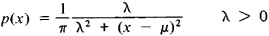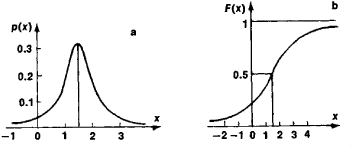Cauchy Distribution

(redirected from Breit-Wigner distribution)

Cauchy distribution

[kō·shē dis·trə′byü·shən]
(statistics)
A distribution function having the form M /[π M 2+ (x-a)2], where x is the variable and M and a are constants. Also known as Cauchy frequency distribution.

Cauchy Distribution

a special type of probability distribution of random variables. Introduced by Cauchy, it is marked by the densityThe characteristic function is

f(t) = exp (μit − λ ǀ t ǀ)

The Cauchy distribution is unimodal and symmetric with respect to the point x = μ, which is its mode and median. NoFigure 1. Cauchy distribution: (a) probability density, (b) distribution function

moments of positive order of a Cauchy distribution exist. Figure 1 depicts a Cauchy distribution for μ = 1.5 and λ = 1.

Mentioned in ?
References in periodicals archive ?
More specifically it was assumed that the Breit-Wigner distribution of the mass value could not be translated in a deterministic, unique and logical way, using the Standard Model, into the exponential decay time distribution that we observe.
r] and the exponential and Breit-Wigner distributions

Site: Follow: Share:
Open / Close